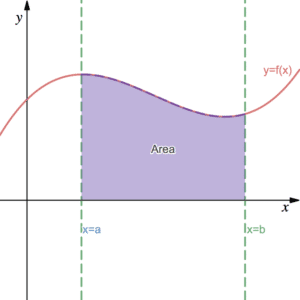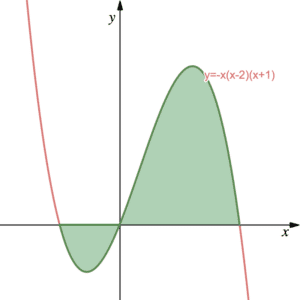# Definite Integrals

Definite integrals are those integrals that have limits. They are particularly useful for finding areas beneath curves. Before exploring this page and the concept of Definite Integrals, read Integration Fundamentals. We recommend that you are familiar with the First Fundamental Theorem of Calculus.

## The Fundamental Theorem of Calculus - Part 2

The Fundamental Theorem of Calculus is split into two parts. The formal definitions are too advanced for this page but we will explore what the theorem states loosely. The second part of the theorem, we often call the Second Fundamental Theorem of Calculus, relates to definite integration. See An Introduction to Integration for the first part of the theorem.

### Definite Integrals

Definite integrals are integrals that have scalar limits (single number limits). Limits appear in pairs – an upper/lower limit to the right of the top/bottom of the integral. Integrals with limits are written in the form as follows:

$\int_a^b f(x)\, dx$

The Second Fundamental Theorem of Calculus states that we can evaluate this integral as the difference between the antiderivative (or indefinite integral) evaluated at the given limits:

$\int_a^b f(x) \, dx=F(b)-F(a)$.

Here, $F(x)$ is the antiderivative of $f(x)$, that is to say $F'(x)=f(x)$. We find the antiderivative $F(x)$ by integrating $f(x)$. Note that when evaluating definite integrals, it is not necessary to include a constant of integration as it always disappears when substituting in the limits – see Example 1. Remind yourself of what a constant of integration is here. Also note that $F(b)-F(a)$ is sometimes written as $\left[F(x)\right]_a^b$ – see Example 2.

Note that the constant of integration always disappears in this way and so is typically left out when performing definite integration.

## Finding an area beneath a curve using definite integralsThe integral expression above: $\int_a^b f(x)\, dx$, has a physical interpretation. It represents the area beneath the graph of $y=f(x)$, above the $x$-axis and between the lines $x=a$ and $x=b$ as we can see in the diagram. Click here for a more formal explanation of the integral and how integral expressions represent areas. Note that if the curve is below the $x$-axis, then the integral will be negative – we must be careful when interpreting ‘negative areas’.

Exam questions may expect you to use definite integration to find the area beneath a curve.  It is important to remember that, in some questions, you may have to evaluate some areas separately if some of the curve is below the $x$-axis – see Example 3. In other questions they may expect you to find the area between a curve and a straight line in a variety of different scenarios – see Example 4 for one such scenario.

In more advanced levels of mathematics, a question may ask you to find the area between two curves – see Example 5.

## Examples of Definite Integrals

Evaluate $\int_1^4 2x^3\, dx$

Evaluate $\int_4^9\left(6x^{\frac{1}{2}}-5x^{\frac{3}{2}}\right)\, dx$

The diagram shows the curve with equation $y=-x(x-2)(x+1)$. Find the exact area shaded shown in green.The diagram shows the curve $y=x^2$ and the straight line $y=0.5x+2$. Find the shaded area shown in purple to 3 decimal places.

(Advanced Maths such as Year 2 A-Level)

Find the area bounded by the curve $y=9-x^2$ and $y=x^2-7x+12$.